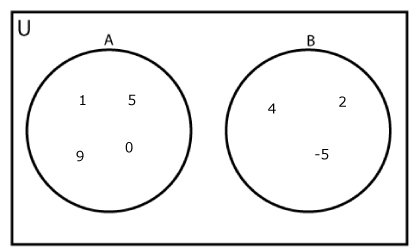# Python Set isdisjoint()

The isdisjoint() method returns True if two sets are disjoint sets. If not, it returns False.

Two sets are said to be disjoint sets if they have no common elements. For example:

```A = {1, 5, 9, 0}
B = {2, 4, -5}```

Here, sets A and B are disjoint sets.The syntax of isdisjoint() is:

`set_a.isdisjoint(set_b)`

## isdisjoint() Parameters

The isdisjoint() method takes a single argument (a set).

You can also pass an iterable (list, tuple, dictionary and string) to disjoint(). The isdisjoint() method will automatically convert iterables to set and checks whether the sets are disjoint or not.

## Return Value from isdisjoint()

The isdisjoint() method returns

• `True` if two sets are disjoint sets (if set_a and set_b are disjoint sets in above syntax)
• `False` if two sets are not disjoint sets

## Example 1: How isdisjoint() works?

```A = {1, 2, 3, 4}
B = {5, 6, 7}
C = {4, 5, 6}

print('Are A and B disjoint?', A.isdisjoint(B))
print('Are A and C disjoint?', A.isdisjoint(C))
```

When you run the program, the output will be:

```Are A and B disjoint? True
Are A and C disjoint? False```

## Example 2: isdisjoint() with Other Iterables as arguments

```A = {'a', 'b', 'c', 'd'}
B = ['b', 'e', 'f']
C = '5de4'
D ={1 : 'a', 2 : 'b'}
E ={'a' : 1, 'b' : 2}

print('Are A and B disjoint?', A.isdisjoint(B))
print('Are A and C disjoint?', A.isdisjoint(C))
print('Are A and D disjoint?', A.isdisjoint(D))
print('Are A and E disjoint?', A.isdisjoint(E))
```

When you run the program, the output will be:

```Are A and B disjoint? False
Are A and C disjoint? False
Are A and D disjoint? True
Are A and E disjoint? False```